Courses

# Short & Long Answer Question: Thermal Properties of Matter- 3 Notes | EduRev

## Class 11 : Short & Long Answer Question: Thermal Properties of Matter- 3 Notes | EduRev

The document Short & Long Answer Question: Thermal Properties of Matter- 3 Notes | EduRev is a part of the Class 11 Course Physics Class 11.
All you need of Class 11 at this link: Class 11

Q.101 Can a gas be liquified at any temperature by the increase of pressure alone?

Answer: No. A gas can be liquified by pressure alone, only when its temperature is below its critical temperature.
Q.102 What is the effect of pressure on melting point of a solid?

Answer: The melting point of a solid may increase or decrease depending on the nature of solid. For solids such as ice which contracts on melting, it is lowered while for solids such as sulphur and wax which expand on melting it increases.
Q.103 How does the boiling point of water change with pressure?

Answer: The boiling point of water increases with the increase in pressure.
Q.104 What is the temperature above which steam will not condense to water even if it is compressed (isothermally) to very large pressure?

Answer: Above the critical temperature (374.1C for water), steam will not condense to water.
Q.105 What is the significance of negative slope of ice line of water?

Answer: It indicates that the melting point of ice decreases with increase in pressure (on ice line). This is because volume of water formed on melting is less than the volume of ice (before melting).
Q.106 Are the relative amounts of ice, water and vapour fixed at the triple point water?

Answer: At the triple-point of water, the temperature and pressure are fixed. However, the relative amounts of the three phases are not unique. The relative amounts of the three phases can be varied by adding or taking out heat from the system.
Q.107 What happens if water vapour at a pressure of 0.004 atm is cooled to 0C?

Answer: The pressure corresponding to triple point is 610 Pa which is equal to nearly 0.006 atm. It follows from the P-T phase diagram of water that at a pressure lower than this pressure, water vapour condenses directly to ice without passing through the liquid phase.
Q.108 Water exists in liquid phase at 30C at 1 atmospheric pressure. How would you convert this water to vapour form without increasing its temperature?

Answer: Water at 30C can be converted into vapour by reducing its pressure until it equals the vapour pressure of water at 30C. This route is along a vertical line on the P-T diagram, with T = 30C.
Q.109 What are the critical temperature and pressure for CO2? What is their significance?

Answer: The critical temperature and pressure of CO2 are 31.1C and 73.0 atm respectively. Above this temperature, CO2 will not liquify even if compressed to high pressures.
Q.110  Ice of 0 is converted into steam at 100° C. State the isothermal changes in the process.

Answer: The isothermal changes are (i) Conversion of ice at 0C into water at 0C. (ii) Conversion of water at 100C into steam at 100C.

Q.111 Explain why a new quilt is warmer than an old one.

Answer: A new quilt contains more air in its pores as compared to the old quilt. As air is bad conductor of heat, it does not allow heat to be conducted away from our body to the surroundings and we feel warmer in it.
Q.112 Give reasons why water is considered unsuitable for use in thermometers.

Answer: Water is considered unsuitable for use in thermometers due to following reasons:

(i) The expansion of water with temperature is non-uniform.

(ii) Due to its large specific heat and low thermal conductivity, a water thermometer does not respond to changes in temperature quickly.

(iii) Water is invisible, sticks to glass and has high rate of evaporation.

(iv) Its temperature range is small from 0C to 100C
Q.113 Give four reasons why is mercury used in thermometers.

Answer: The reasons for using mercury in a thermometer are

(i) Mercury has a uniform expansion over a wide range of temperature.

(ii) Mercury is opaque and bright, so it can be easily seen in a glass tube.

(iii) It does not stick to the walls of the glass tube.

(iv) It is a good conductor of heat and has low thermal capacity.
Q.114 Give reasons why is a platinum wire used in a resistance thermometer.

Answer: The reasons for using a platinum wire in a resistance thermometer are:

(i) The resistance of a platinum wire increases uniformly with the rise in temperature (from 200C to 1200C).

(ii) It does not react chemically with other substances

(iii) Its melting point is quite high (1800C).
Q.115 Give some merits of gas thermometers over those of mercury thermometers.

Answer: Some merits of gas thermometers are

(i) A gas thermometer is more sensitive than a mercury thermometer,

(ii) The working of a gas thermometer is independent of the nature of the gas used.

(iii) A gas thermometer can measure very low and very high temperatures.

Q.116 Name the suitable thermometers to measure the following temperatures: - 80 C, 60 C,  250∘ C, 780 C, 2000 C

Answer: Gas thermometer for -80C. Mercury thermometer for 60C. Platinum resistance thermometer for 250C and 780C. Total radiation pyrometer for 2000C.
Q.117 Suggest suitable methods for measuring the temperature (i) surface of the sun, (ii) surface of the earth, (iii) an insect, and (iv) liquid helium.

Answer: (i) By using total radiation pyrometer. (ii) By using a thermoelectric thermometer by embedding its hot junction in the earth. (iii) By using a thermoelectric thermometer by touching its hot junction with the insect. (iv) By using a magnetic thermometer which is based on Curie's law: the susceptibility of a paramagnetic material varies inversely with its absolute temperature.
Q.118 In problem 7, will the distance between the two holes increase or decrease on heating?
Answer:
When the metal sheet is heated, it expands a whole. Therefore, the holes will increase in diameter as well as move outwards. The distance BC between the two holes increases.
Q.119 There are two spheres of same radius and material at same temperature but one being solid while the other hollow. Which sphere will expand more if (i) they are heated to the same temperature (ii) same amount of heat is given to each of them?

Answer: (i) As thermal expansion of isotropic solids is similar to true photographic enlargement, the expansion of a cavity is same as if it were a solid body of the same material i.e.,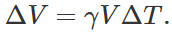.As here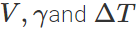are same for both solid and hollow spheres, so the expansions of both will be equal. (ii) If same amount of heat is given to the two spheres, then due to lesser mass, rise in temperature of hollow sphere will be more (as ΔT=Q/Mc) and hence the expansion will be more as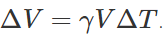Q.120 Two bodies of specific heats c1 and c2 having same heat capacities are combined to form a single composite body. What is the specific heat of the composite body?

Answer: As the heat capacities are equal, so m1c1=m2c2. Let c be the specific heat of the composite body. Then (m1+m2)c=m1c1+m2c2=m1c1+m1c1=2m1c1 or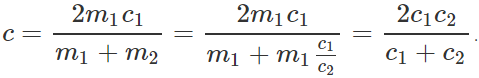Q.121 Two rods A and B are of equal length. Each rod has its ends at temperatures T1 and T2 . What is the condition that will ensure equal rates of flow of heat through the rods A and B?

Answer: Let x be the length of each rod. The rates of flow of heat through the rods A and B will be equal if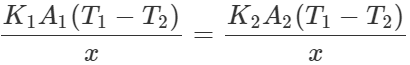or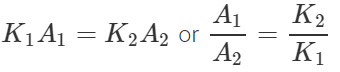Hence for equal rates of flow of heat, the areas of cross-section of the two rods should be inversely proportional to their coefficients of thermal conductivity.
Q.122 Two vessels of different materials are identical in size and wall-thickness. They are filled with equal quantities of ice at 0 C. If the ice melts completely in 10 and 25 minutes respectively, compare the coefficients of thermal conductivity of the materials of the vessels.

Answer: Let K1 and K2 be the coefficients of thermal conductivity of the materials and t1 and t2 be the times in which ice melts in the two vessels. As the same quantity of ice melts in the two vessels, the quantity of heat flowed into the vessels must be same. ∴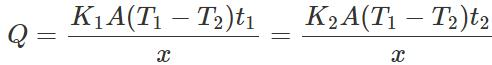or K1t1=K2t2 ∴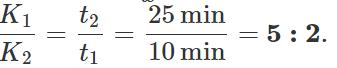Q.123 Two vessels A and B of different materials but having identical shape, size and wall- thickness are filled with ice and kept at the same place. Ice melts at the rate of 100gmin−1 and 150gmin−1 in A and B respectively. Assuming that heat enters the vessels through the walls only, calculate the ratio of thermal conductivities of their materials.

Answer: Let m1 and m2 be the masses of ice melted in same time t (=1 min)in vessels A and B respectively. Then the amounts of heat flowed into the two vessels will be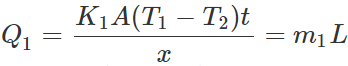?(i)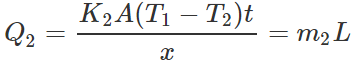?(ii) where L is latent heat of ice. Dividing (i) by (ii), we get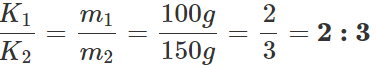Q.124 A sphere, a cube and a thin circular plate, all made of the same material and having the same mass are initially heated to a temperature of 200C.  Which of these objects will cool fastest and which one slowest when left in air at room temperature? Give reasons.

Answer: The thin circular plate has the largest surface area. The sphere has the smallest surface area. Thus the plate will radiate maximum heat while the sphere will radiate minimum heat. Hence the plate will cool fastest and the sphere will cool slowest.
Q.125 There are two rods of the same metal, same length, same area of cross-section, but one of square cross-section and the other of circular cross- section. One end of each is kept immersed in steam. After the steady state is reached, the other ends of the rods are touched. Which one will be hotter? Give reason.

Answer: The surface area of the rod of circular cross-section will be smaller, because for a given area a circle has least perimeter. So the loss of heat by radiation will be small. Hence the other end of the circular rod will be hotter than the other end of the square rod.
Q.126 A solid sphere of copper of radius R and a hollow sphere of the same material of inner radius r and outer radius R are heated to the same temperature and allowed to cool in the same environment. Which of them starts cooling faster?

Answer: Rate of loss of heat by any sphere,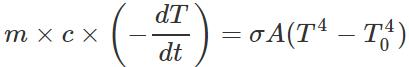Now σ,A,(T4−T40) are same for both the spheres, so the rate of cooling,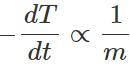Since the hollow sphere has less mass, its rate of cooling will be faster.
Q.127 On a hot day, a car is left in sunlight with all the windows closed. After some time, it is found that the inside of the car is considerably warmer than the air outside. Explain, why.

Answer: Glass transmits about 50% of heat radiation coming from a hot source like the sun but does not allow the radiation from moderately hot bodies to pass through it. Due to this, when a car is left in the sun, heat radiation from the sun gets into the car but as the temperature inside the car is moderate, they do not pass back through its windows. Hence, inside of the car becomes considerably warmer.
Q.128 How does tea in a thermos flask remain hot for a long time?

Answer: The air between the two walls of the thermos flask is evacuated. This prevents heat loss due to conduction and convection. The loss of heat due to radiation is minimized by silvering the inside surface of the double wall. As the loss of heat due to the three processes is minimized, the tea remains hot for a long time.

Q.129 Distinguish between conduction, convection and radiation.

Answer:

 Conduction Convection Radiation 1. Material medium is required. Material medium is required. No material medium is required. 2. It is due to temperature difference. Heat flows from high temperature region to low temperature region. It is due to difference in density. Heat flows from low density region to high density region. It occurs from all bodies at temperatures above 0 K. 3. It occurs in solids through molecular collisions, without actual flow of matter. It occurs in fluids by actual flow of matter. It can take place at large distances and does not heat the inter-  vening medium. 4. It is a slow process. It is also a slow process. It propagates at the speed of light. 5. It does not obey the laws of reflection and refraction. It does not obey the laws of reflection and refraction. It obeys the laws of reflection and refraction.

Q.130 A blackened platinum wire, when gradually heated, first appears dull red, then blue and finally white. Explain why.

Answer: According to Wien's displacement law, when blackened platinum wire is gradually heated, it first emits radiations of longer wavelengths, so it appears red. At higher temperatures, it emits blue radiations more strongly than red and appears blue. At very high temperatures, it emits all radiations strongly and appears white.
Q.131 In a coal fire, the pockets formed by coals appear brighter than the coals themselves. Is the temperature of such a pocket higher than the surface temperature of a glowing coal?

Answer: The temperature of pockets formed by coals are not appreciably different from the surface temperatures of glowing coals. However, the pockets formed by coals act as cavities. The radiations from these cavities are black body radiations and so have maximum intensity. Hence the pockets appear brighter than the glowing coals.
Q.132 Answer the following questions : (a) A vessel with a movable piston maintained at a constant temperature by a thermostat contains a certain amount of liquid in equilibrium with its vapour. Does this vapour obey Boyle's law? In other words, what happens when the volume of vapour is decreased? Does the vapour pressure increase? (b) What is meant by 'superheated water' and 'super cooled vapour'? Do these states of water lie on its P-V-T surface? Give some practical applications of these states of water.

Answer: (a) No, the vapour in equilibrium with its liquid does not obey Boyle's law. When the volume of the vapour is decreased by applying pressure, some of the vapours condense into liquid, maintaining the same pressure of the vapour at the given temperature i.e., vapour pressure does not increase when the volume of vapours is decreased.

(b) Superheated water. Water in liquid phase having temperature above the boiling point of water at the given pressure is called superheated water. It is highly unstable  stage. Super cooled vapour. Water in vapour phase having temperature below its boiling point at the given pressure is called super cooled vapour. It is also highly unstable stage. As the above states of water are not equilibrium states, so they do not lie on P-V-T surface of water. Applications. These unstable states of water are used in bubble chamber and cloud chamber for detecting high speed charged particles.

Offer running on EduRev: Apply code STAYHOME200 to get INR 200 off on our premium plan EduRev Infinity!

## Physics Class 11

75 videos|295 docs|152 tests

,

,

,

,

,

,

,

,

,

,

,

,

,

,

,

,

,

,

,

,

,

;